# ExpectedValueThing .pdf

### File information

Original filename: ExpectedValueThing.pdf

This PDF 1.5 document has been generated by TeX / MiKTeX pdfTeX-1.40.17, and has been sent on pdf-archive.com on 14/08/2016 at 23:20, from IP address 104.254.x.x. The current document download page has been viewed 397 times.
File size: 95 KB (5 pages).
Privacy: public file

ExpectedValueThing.pdf (PDF, 95 KB)

### Document preview

Expected number of tosses to get x heads in a row with a coin
that lands heads with probability p.

OK, so what we are interested in is a number of tosses E after which we can expect a
sequence of x heads in a row, given that the specific coin we are tossing lands on heads
with a probability p. We will end with an equation of the form:
Ex,p = f (p, x)

To get a better intuition for this problem I’m going to think about a specific case
first and slowly make it general. Let’s say we have a fair coin p = 21 and we’re trying to
get 5 heads in a row. We can get a valid equation involving the expected value just by
considering some conditions for success or failure.

Sequence of flips encountered Change in expected number of flips, E
T
Keep flipping: E + 1
HT
Keep flipping: E + 2
HHT
Keep flipping: E + 3
HHHT
Keep flipping: E + 4
HHHHT
Keep flipping: E + 5
HHHHH
Done! E = current number of flips

Because each coin toss is independent, whenever we get a sequence where we fail like
T, we have the same exact chance of flipping heads 5 times in a row again after that flip.
So our expected number of flips after flipping T is still E, but remembering we just made
a flip, we can expect to get 5 heads in E + 1 flips total. We can extend this thinking to
all of the failing coin flip sequences.
For HHHHH, we know how many coin flips are needed to get 5 in a row, because we
have just flipped them—E = 5.

1

Now, we can think about how often each of these possibilities occur, and use it to get
an expression that we can then move around to get E in terms of p and x.

Probability of happening Seq. of flips encountered Total num. of flips needed
1
T
E+1
2
1
HT
E+2
4
1
HHT
E+3
8
1
HHHT
E+4
16
1
HHHHT
E+5
32
1
HHHHH
5
32
Now, we can add up all of these expected numbers of flips multiplied by their probability of occurring to give us our total E.
E = 21 (E + 1) + 14 (E + 2) + 18 (E + 3) +

1
16 (E

+ 4) +

1
32 (E

+ 5) +

1
32 (5)

Then it’s just algebra the rest of the way home.
1
1
1
1
1
1
E = (E + 1) + (E + 2) + (E + 3) + (E + 4) + (E + 5) + (5)
2
4
8
16
32
32
E=
E−

31
62
E+
32
32

31
62
E=
32
32

E(1 −

31
62
)=
32
32

E(

1
62
)=
32
32
E = 62

So now we have a little bit of background that will help us think of the more general
case. How could we generalize the equation we got for E for any probability p and any

2

Thinking about the sequence of tosses that got us each of the probabilities for the
fair coin will help us to find all of the likelihoods of a certain sequence happening for
a coin that has chance p of landing on heads. If p is the chance we get heads, then
(1 − p) is the chance we get tails. So, finding the probability of any sequence is as easy
as substituting those probabilities in for the specific sequence of tosses we want. For
example, the probability we get HHHT would be p ∗ p ∗ p ∗ (1 − p). Extending that
principle we can say:

Probability of happening Seq. of flips encountered Total num. of flips needed
(1 − p)
T
E+1
p(1 − p)
HT
E+2
2
p (1 − p)
HHT
E+3
p3 (1 − p)
HHHT
E+4
4
p (1 − p)
HHHHT
E+5
5
p
HHHHH
5

Alright, we’ve generalized for any sort of coin weighted to land on heads or tails more
frequently. Let’s see what the formula would look like in this instance (using summation
notation to simplify the terms with E in them).
E=

5
X

pk−1 (1 − p)(E + k) + 5p5

k=1

All that’s left now is to see how the formula would change when we define success
as any arbitrary length of heads x. For any length we would need to account for all
the failing sequences from T through HHH
. . . HT}, and lastly the sequence we defined as
|
{z
x

success HHH
| {z. . . H}.
x

The probability of the sequence happening and the number of flips you need for success when each of the sequences occurs both follow directly from these general length
sequences:

3

Probability of happening Seq. of flips encountered
(1 − p)
T
p(1 − p)
HT
2
p (1 − p)
HHT
···
···
px−1 (1 − p)
HHH . . . HT
x
p
HHH . . . HH

Total num. of flips needed
E+1
E+2
E+3
···
E+x
x

Finally we’re left with:
E=

x
X

pk−1 (1 − p)(E + k) + xpx

k=1

All that’s left now is more algebra and a few tricks involving summation.

x

E = xp +

x
X

pk−1 (1 − p)(E + k)

k=1
x

E = xp + (1 − p)
E = xpx + (1 − p)
E = xpx + (1 − p)

x
X

pk−1 (E + k)

k=1
x
X

pk−1 E +

k=1
x
X

E
p

k=1

pk +

x
X

!
pk−1 k

k=1
x
X
k−1

p

!
k

k=1

At this junction, I use the fact that:
x
X
k=1

x
X
1 − px+1
1 − px
xpx
− 1 and that
pk−1 k =

p =
1−p
(1 − p)2 (1 − p)
k

k=1

4

So we are left with:
E = xpx + (1 − p)

E = xpx +

E = xpx +

1 − px
(1 − p)

E(1 − (1 − px )) =

1 − px
(1 − p)

E(px ) =

1 − px
(1 − p)

1−p
−1
1−p

!
+

E
p − px+1
p

!

x

+

x

x

1 − px
− xpx
(1 − p)

!!

xp
1−p

(1 − p)2 (1 − p)

!
E
(1 − px+1 ) − (1 − p) +
p

E = E(1 − px ) +
E − E(1 − px ) =

E
p

x−1

1−p
− xpx
(1 − p)

!!

!

1 − px
(1 − p)

And so we finish with our expected length in terms of p and x:
1 − px
Ex = x
p (1 − p)

From a problem I got from Math Stack Exchange:
-/u/janoseye

5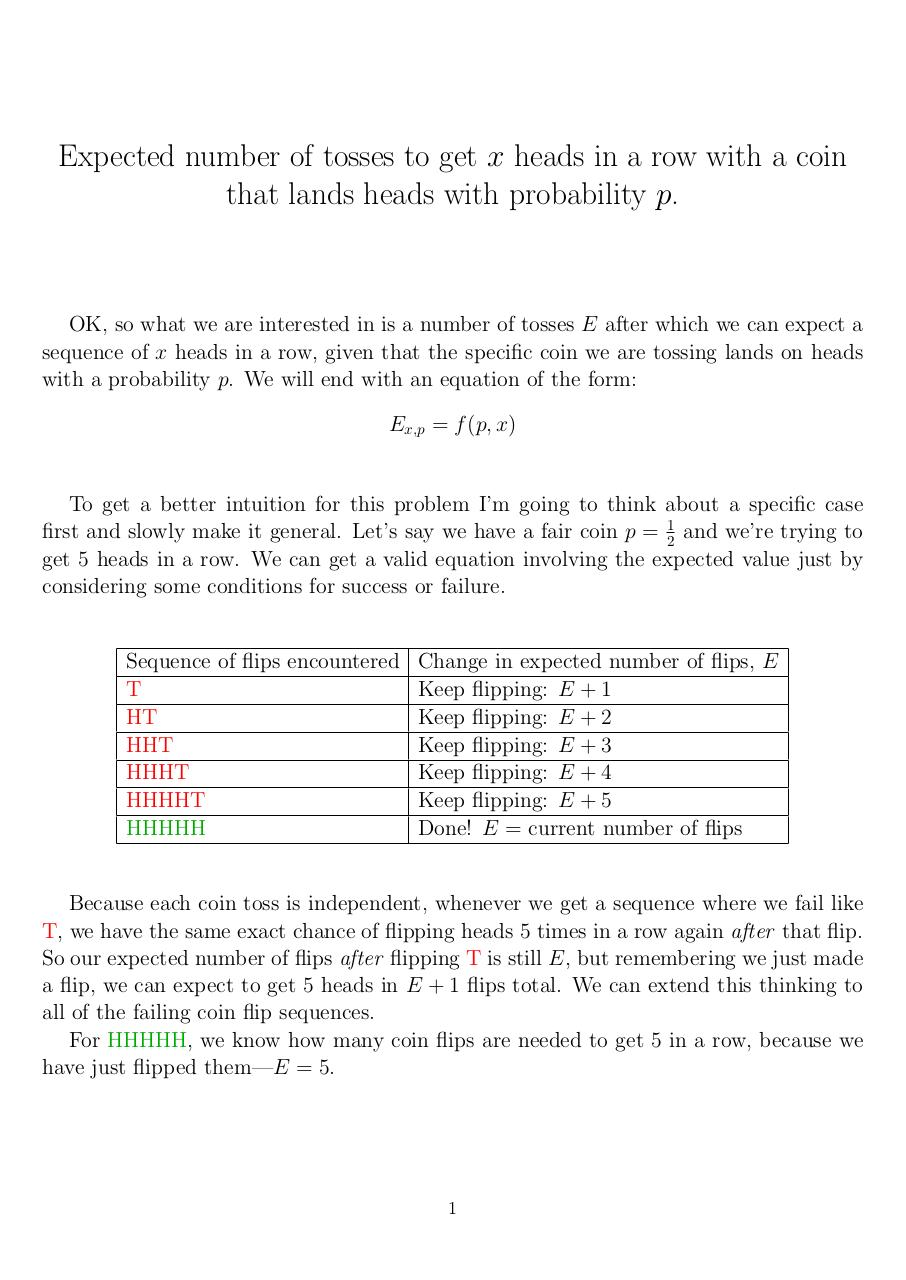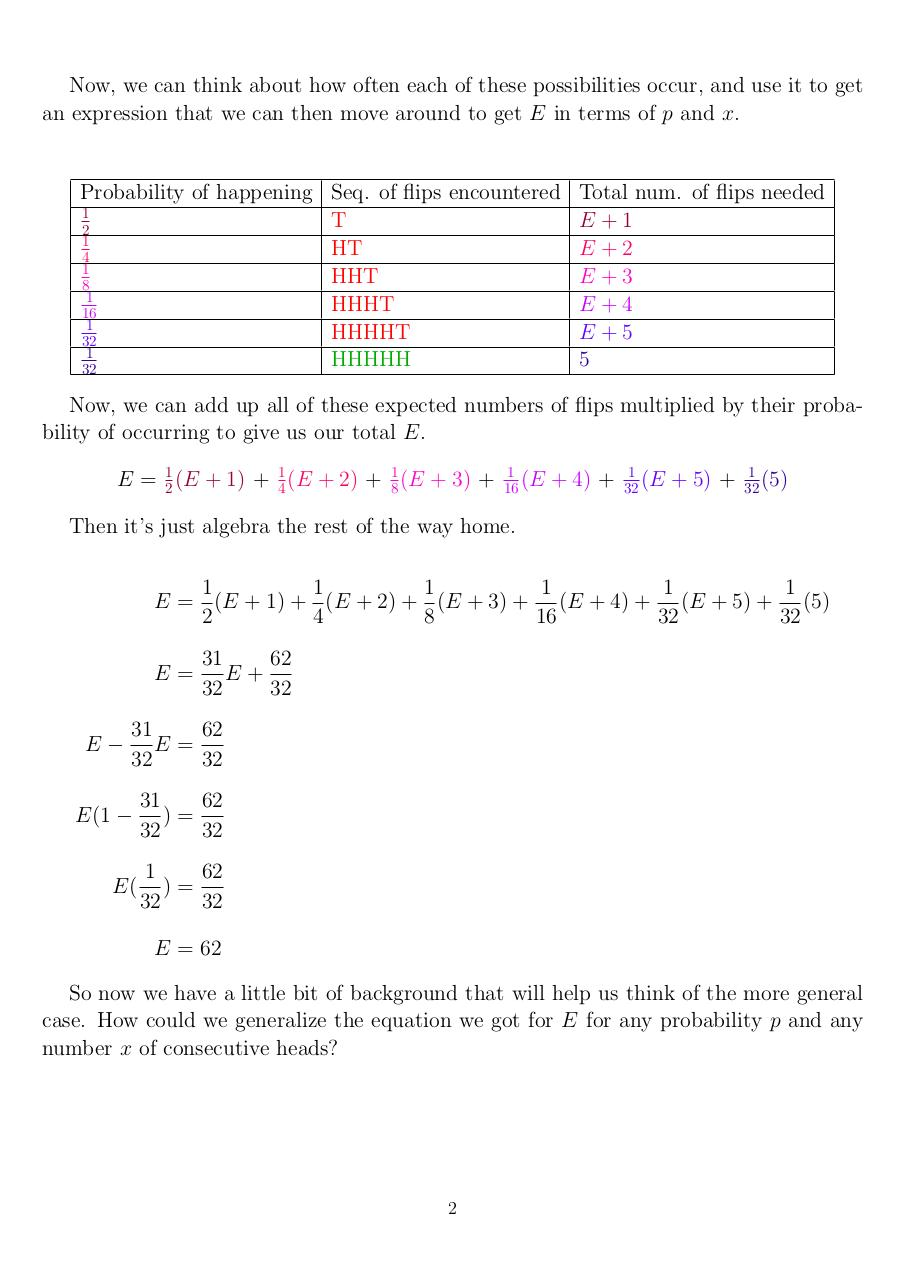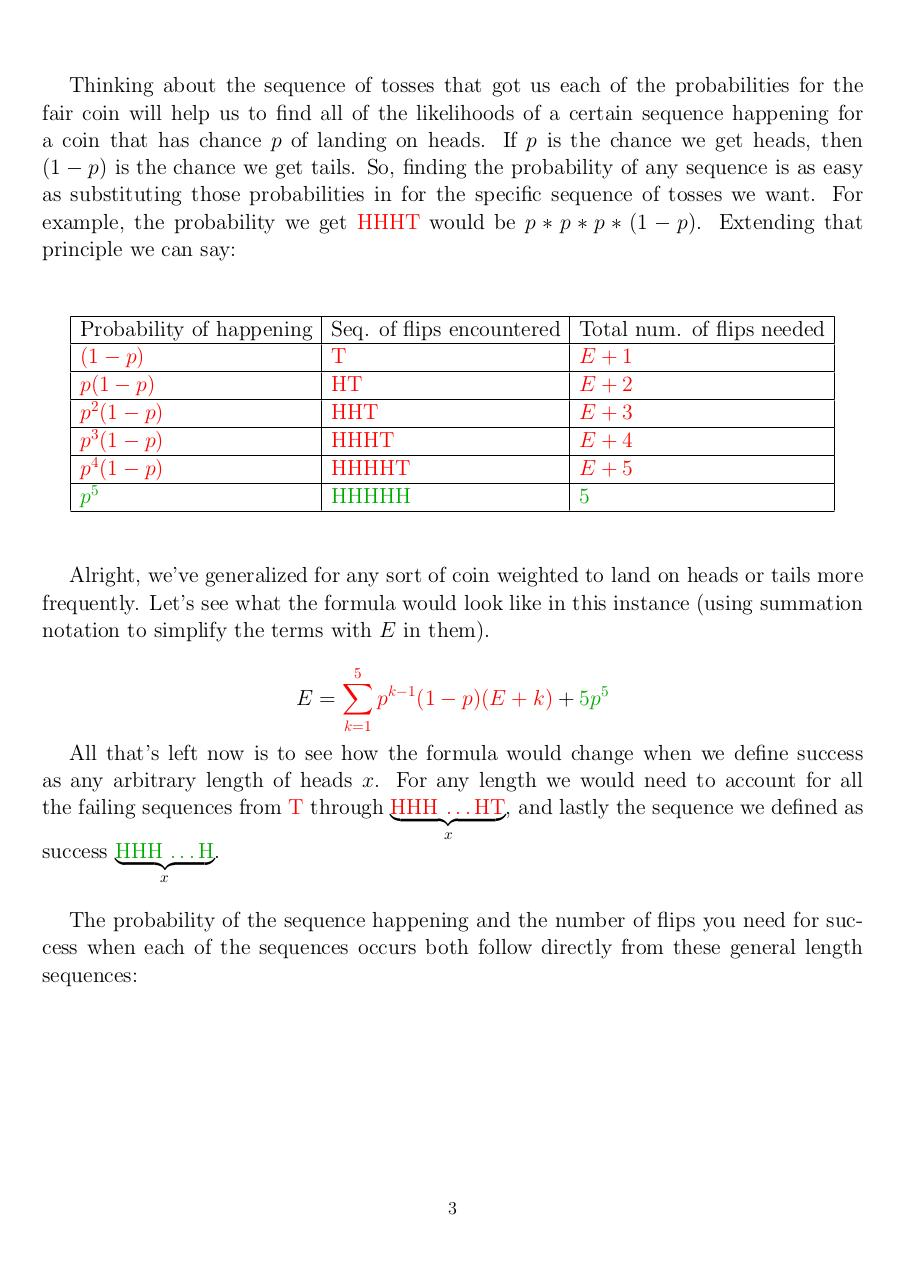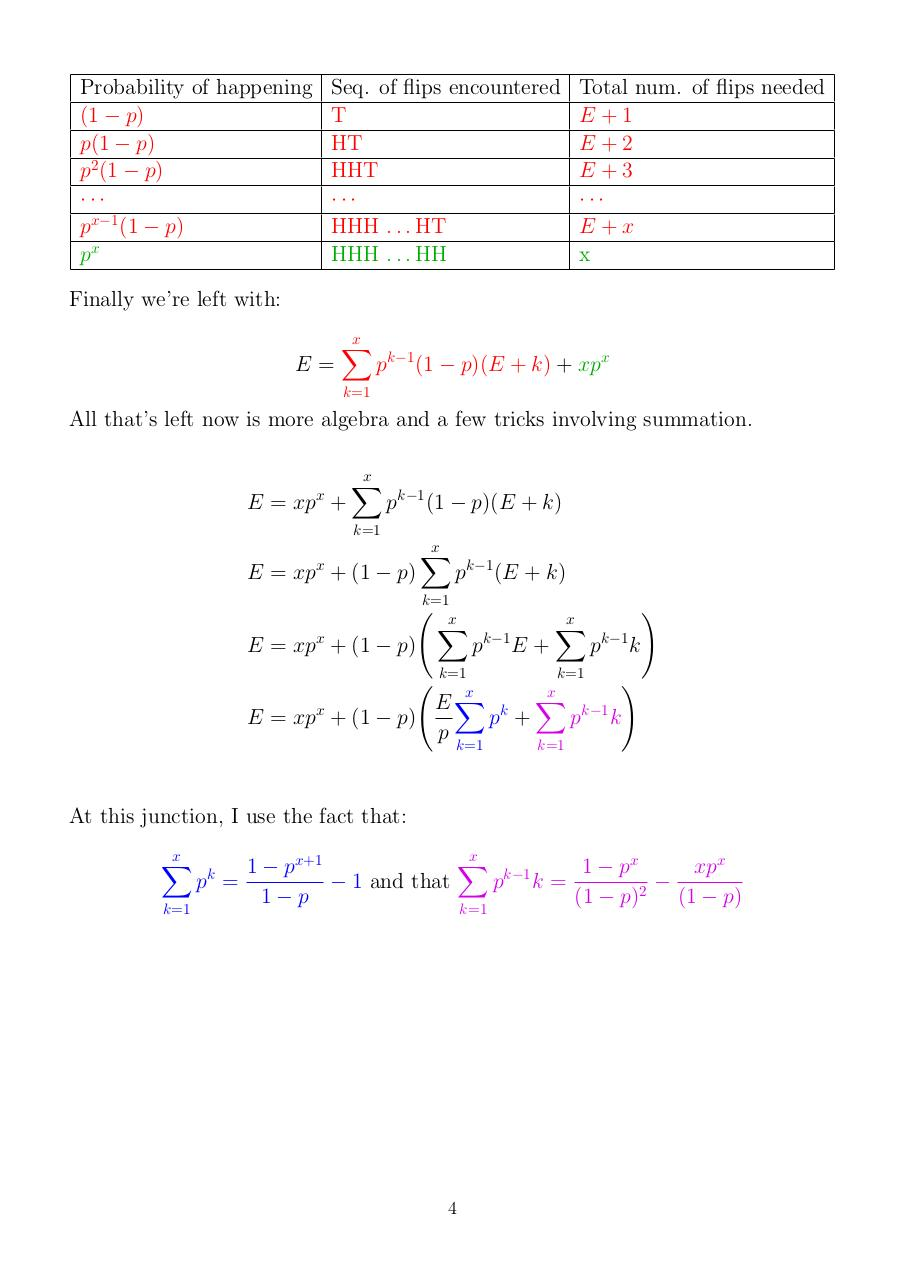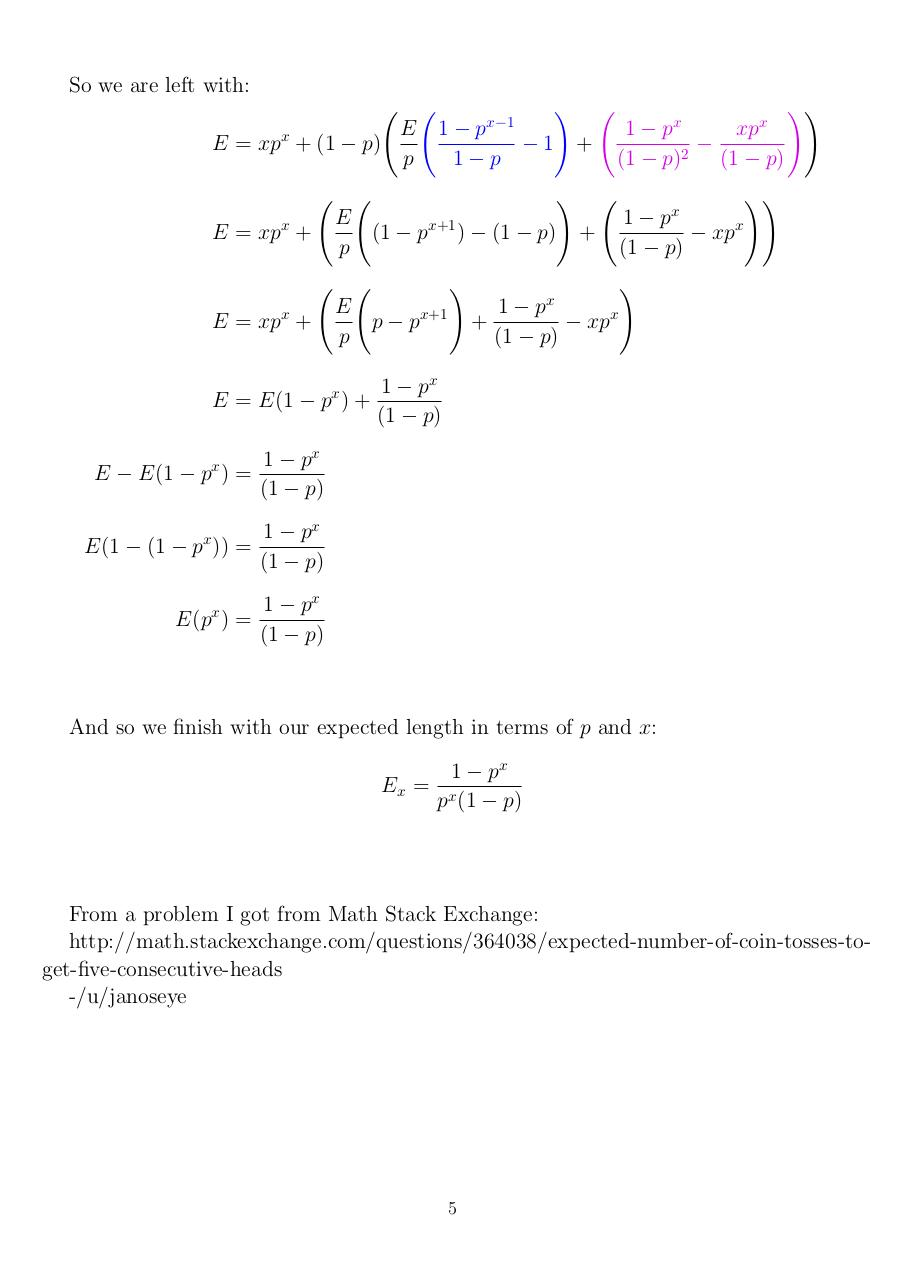#### HTML Code

Copy the following HTML code to share your document on a Website or Blog

#### QR Code### Related keywords